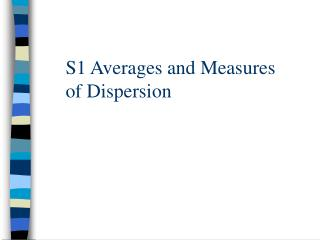# S1 Averages and Measures of Dispersion - PowerPoint PPT PresentationDownload PresentationS1 Averages and Measures of Dispersion

S1 Averages and Measures of DispersionDownload Presentation## S1 Averages and Measures of Dispersion

- - - - - - - - - - - - - - - - - - - - - - - - - - - E N D - - - - - - - - - - - - - - - - - - - - - - - - - - -
##### Presentation Transcript

1. S1 Averages and Measures of Dispersion

2. S1 Measures of Dispersion Objectives: To be able to find the median and quartiles for discrete data To be able to find the median and quartiles for continuous data using interpolation

3. Can you work out the rule for finding median and quartiles from discrete data? • LQ Median UQ • 1 2 3 4 5 1.5 3 4.5 • 1 2 3 4 5 6 • 1 2 3 4 5 6 7 • 1 2 3 4 5 6 7 8 • 1 2 3 4 5 6 7 8 9 • Can you spot any rules for n amount of numbers in a list?

4. LQ  n/4 If n/4 is a whole number find the mid point of corresponding term and the term aboveIf n is not a whole number, round the number up and find the corresponding term UQ  3n/4 If 3n/4 is a whole number find the mid point of corresponding term and the term aboveIf n is not a whole number, round the number up and find the corresponding term

5. Median  n/2 If n/2 is a whole number find the midpoint of the corresponding term and the term above If n/2 is not a whole number, round up and find the corresponding term

6. Calculate the mean, median and inter quartile range from a table of discrete data Mean = Σfx Σf

7. Calculate the mean, median and inter quartile range from a table of discrete data Median = n/2 Cumulative frequency Median = 95/2 = 47.5 = 48th value 3 20 49 83 95 Median = 37 CDs LQ = 95/4 = 23.75 LQ = 24th value LQ (Q1) = 37 CDs UQ (Q3) = 95/4 x 3 = 71.25 UQ = 72nd value UQ (Q3) = 38 CDs IQR = Q3-Q1 = 38-37=1

8. Calculate the mean, median and inter quartile range from a table of continuous data Median = n/2 We do not need to do any rounding because we are dealing with continuous data Cumulative frequency 2 27 57 70 Median = 70/2 = 35th value This lies in the 34-36 class but we don’t know the exact value of the term

9. Using interpolation to find an estimate for the median 33.5mm m 36.5mm 35 27 57 m – 33.5 = 8 3 30 m – 33.5 = 35 - 27 36.5 – 33.5 = 57 - 27 m – 33.5 = 0.26 x 3 m = 33.5 + 0.8 = 34.3

10. Using interpolation to find an estimate for the lower quartile LQ = 70/4 = 17.5 (in the 32-33 group) 31.5mm Q1 33.5mm 17.5 2 27 Q1 – 31.5 = 15.5 2 25 Q1 – 31.5 = 17.5 - 2 33.5 – 31.5 = 27 - 2 Q1 – 31.5 = 0.62 x 2 Q1 = 31.5 + 1.24 = 32.74

11. Using interpolation to find an estimate for the upper quartile UQ = 70/4x3 = 52.5 (in the 34-36 group) 33.5mm Q3 36.5mm 52.5 27 57 Q3 – 33.5 = 25.5 3 30 Q3 – 33.5 = 52.5 - 27 36.5 – 33.5 = 57 - 27 Q3 – 33.5 = 0.85 x 3 Q1 = 33.5 + 2.55 = 36.05

12. Median = LB + ½n – fB x w fU - fB LQ = LB + ¼n – fB x w fU - fB UQ = LB + ¾n – fB x w fU - fB Summary of rules n = total frequency w = class width fB = cumulative frequency below median/lq/uq fU = cumulative frequency above median/lq/uq

13. Q1=74.5 + 500-250 x 5 738-250 Q1=77.06 Q2=79.5+1000-738 x 5 1370-738 Q2=81.57 Q3=84.5+1500-1370 x 5 1840-1370 Q3=85.88 The lengths of a batch of 2000 rods were measured to the nearest cm. The measurements are summarised below. Cumulative frequency 11 60 250 738 1370 1840 1977 2000 By altering the formula slightly can you work out how to find the 3rd decile (D3) and the 67th percentile (P67)?

14. D3=74.5 + 600-250 x 5 738-250 D3=78.09 Answers P67=79.5 + 1340-738 x 5 1370-738 P67=84.26